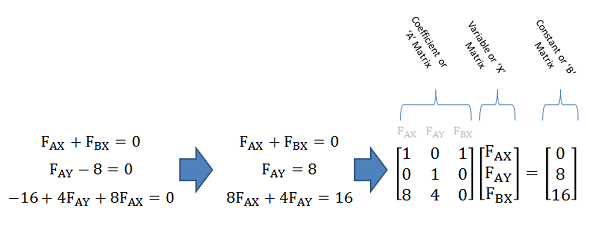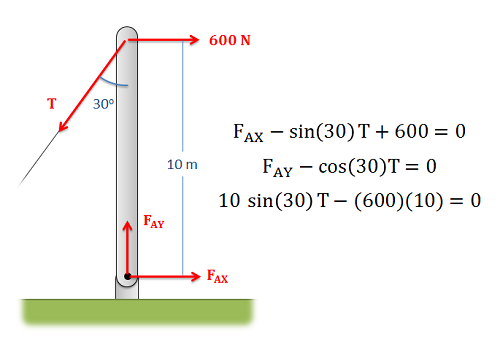﻿ Mechanics Map - Matrix Equations

# Solving Systems of Equations with Matrices

A system of equations, is any set of equations that share some variables. A linear equation is an equation that consists entirely of constants and simple variables. These variables can only be multiplied by a constant, and cannot be multiplied together, raised to an exponent, used on logs or square roots, or use other more complex mathematical functions. An example of a system of linear equations is provided below.

 $F_{AX}+F_{BX}=0$ $F_{AY}-8=0$ $-16+4F_{AY}+8F_{AX}=0$

In courses such as statics and dynamics we will often wind up with a system of linear equations and be asked to solve for the unknowns in those equations. When we have just a few equations in our system, we will generally solve the equations by hand using algebraic methods such as substitution or elimination though addition or subtraction. For larger and more complex problems we can wind up with larger systems of equations and at some point the math may become difficult to handle by hand. For these large systems of linear equations, the easiest way to solve for the unknowns is to convert the system of equations into a single matrix equation, and then use computer tools to solve the matrix equation for unknowns. Some computer tools will let you enter the system of equations manually, but in the background the computer is probably just converting it to a matrix equation in the background. For this reason it can be useful to understand this process.

In terms of assumptions, it is important to mention that this method will only work with systems of linear equations, and to have a solvable matrix equation we will need to have the same number of equations as unknown variables. For example, above we have a system of equations with three equations and three unknown variables. If these numbers do not match we will not be able to solve the matrix equation using the method described below.

## Converting a System of Equations to a Matrix Equation:

The first step in converting a system of equations into a matrix equation is to rearrange the equations into a consistent format. Generally we will put all the variables with their coefficients on one side of the equation and the constants on the other side of the equation. Additionally, it is best to list the variables in the same order in each equation. This process of rearranging the equations will make conversion later on easier.To convert a system of equations into a single matrix equation, we will first rearrange the equations for a consistent order, then we will write out the coefficient (A), variable (X), and constant (B) matrices.

Next we will begin the process of writing out the three matrices that make up the matrix equation. These three matrices are the coefficient matrix (often referred to as the A matrix), the variable matrix (often referred to as the X matrix), and the constant matrix (often referred to as the B matrix).

• The coefficient matrix (or A matrix) is an N x N matrix (where N is the number of equations / number of unknown variables) that contains all the coefficients for the variables. Each row of the matrix represents a single equation while each column represents a single variable (it is sometimes helpful to write the variable at the top of each column). For instances where a variable does not show up in an equation, we assume a coefficient of 0.
• The variable matrix (or X matrix) is a 1 x N matrix that contains all the unknown variables. It is important that the order of the variables in the coefficient matrix match the order of the variables in the variable matrix.
• Finally, on the other side of the equal sign we have the constant matrix (or B matrix). This is a N x 1 matrix containing all the constants from the right side of the equations. It is important that the order of the constants matches the order of equations in the coefficient matrix.

Once we have the three matrices set up we are ready to solve for the unknowns in the variable matrix.

## Solving the Matrix Equation:

Starting with our A, X, and B matrices in the matrix equation below, we are looking to solve for for values of the unknown variables that are contained in our X matrix.

 $[A][X]=[B]$

For a scalar equation, we would simply do this by dividing both sides by A, where the value for X would be B/A. With matrix equation we will instead need to multiple both sides of the equation by the inverse of the A matrix. This will cancel out the A matrix on the left side, leaving only the X matrix that you are looking for. On the left we will have the inverse of the A matrix times the B matrix. The result of this operation will be a 1 x N matrix containing the solution for all the variables. The value in each row of the solution will correspond to the variable listed in the same row of the X matrix.

 $[X]=[A]^{-1}[B]$

It is possible to find the inverse of the A matrix by hand and then multiply this with the B matrix, but this process would take longer than just solving the equations using algebra. The true strength of the method is that computer tools such as MATLAB or Wolfram Alpha can perform the matrix inversion and multiplication for you.

## Worked Problems:

### Question 1:

The equilibrium equations for the body shown below are listed on the right. Convert the system of equations into a single matrix equation and solve for the unknowns.